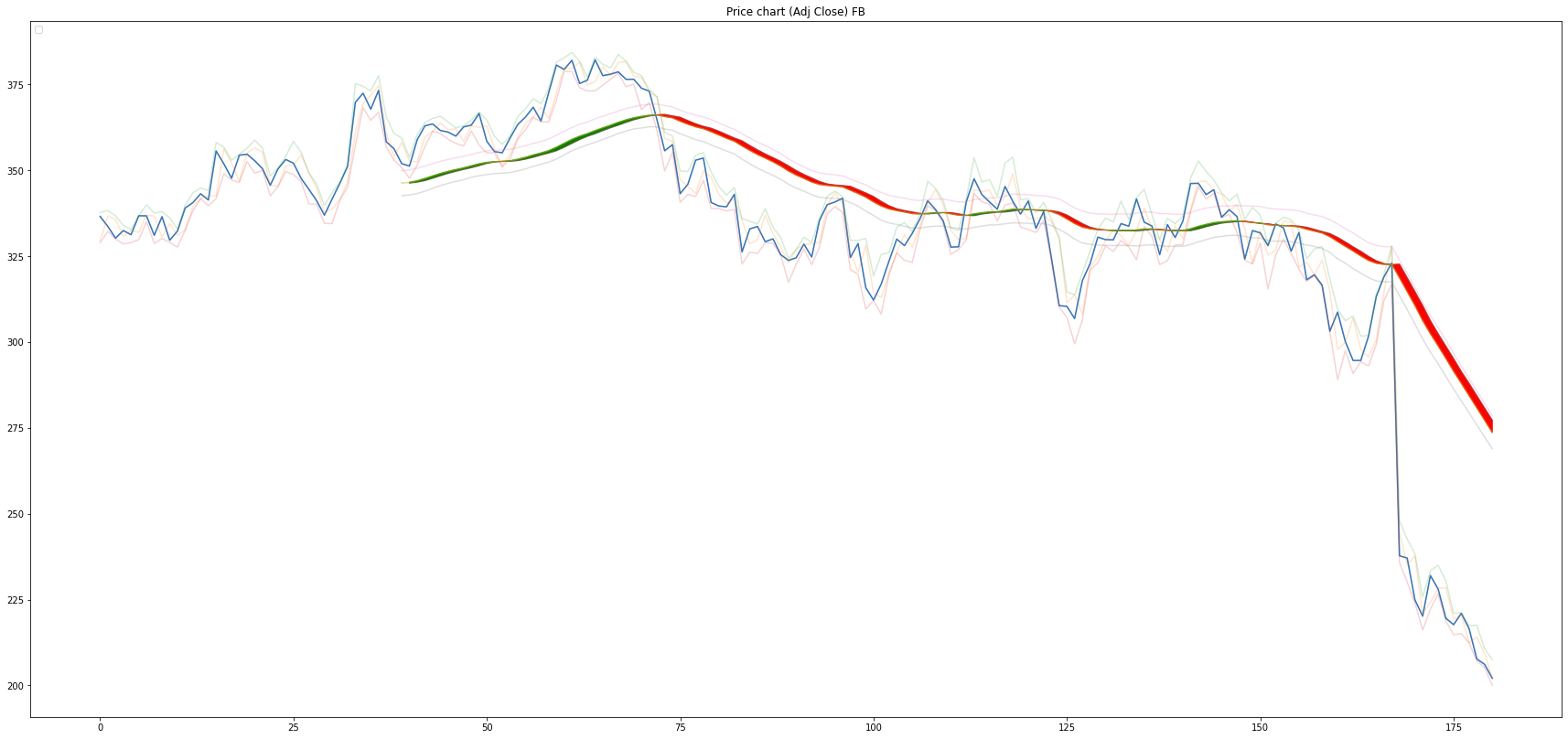## Smoothed Heikin Ashi candlesticks¶

In this article we will discuss alternative usage of Heikin Ashi candlestics (HA) for stocks/crypto analytics and trading in general. Regular Heikin Ashi candlestics make it easier to determine longer term trend by filtering out noise from OHLC price data. If we want to suppress noise further, we can take moving averages (Simple/Exponencial) of OHLC prices to get Smoothed Heikin Ashi candlesticks. It might prove useful to plot both regular OHLC candlesticks alongside (Smoothed/Regular) Heikin Ashi candlesticks.

Heikin Ashi constructed from Moving averages of OHLC prices behave similarly to moving averages. With one benefit - we can plot green/red candle of such HA candlestics to give us info about bullish/bearish price trend. With that said, we can make simplification during visualization - instead of plotting actual candlestics, we will plot HA candlestics as line graph and will color space between the Smoothed Heikin Ashi Open and Close lines accordingly. It will give us pretty much the same info as the actual HA candlestics. For candlestics graphs please check sources at the bottom of the article.

Regular Heikin Ashi: $$C_{ha} = \frac{1}{4}(Open + High + Low + Close)$$ $$O_{ha} = \frac{1}{2}(Open_{i-1} + Close_{i-1})$$ $$H_{ha} = Max[High, Open, Close]$$ $$L_{ha} = Min[Low, Open, Close]$$

Smoothed Heikin Ashi: $$\overline{C_{ha}} = \frac{1}{4}( \overline{Open} + \overline{High} + \overline{Low} + \overline{Close})$$ $$\overline{O_{ha}} = \frac{1}{2}(\overline{Open_{i-1}} + \overline{Close_{i-1}})$$ $$\overline{H_{ha}} = Max[\overline{High}, \overline{Open}, \overline{Close}]$$ $$\overline{L_{ha}} = Min[\overline{Low}, \overline{Open}, \overline{Close}]$$

Optional installs

In :
# uncomment on first run in google colab
#!pip3 install yfinance
#!pip3 install pandas_ta


Imports

In :
import sys
import os

# ___library_import_statements___
import pandas as pd
import pandas_ta as ta # for exponencial moving averages (better than EWM in regular pandas)

# make pandas to print dataframes nicely
pd.set_option('expand_frame_repr', False)

import numpy as np
import matplotlib.pyplot as plt
import datetime
import time

import yfinance as yf


Variables definitions

In :
ticker = 'FB'

start_time = datetime.datetime(2021, 6, 6)
#end_time = datetime.datetime(2022, 1, 1)
end_time = datetime.datetime.now().date().isoformat()     # today

In :
# Heikin Ashi related vars
M=40               # lookback period for averaging OHLC values
#ma_type = 'SMA'   # Simple Moving Average
ma_type = 'EMA'    # Exponencial Moving Average


Function definitions

In :
def get_data(ticker):
# use numerical integer index instead of date
ticker_df = ticker_df.reset_index()
return ticker_df

In :
def construct_df(ticker):
#get data from yahoo API
df = get_data(ticker)
return df

In :
def plot_data_HA(df, markers=False):
# plots price data with Smoothed Heikin Ashi overlay

plt.figure(figsize=(30,14))

plt.title('Price chart (Adj Close) ' + ticker)

# stock OHLC line visualization
plt.plot(df['Open']  , alpha=0.2)
plt.plot(df['High']  , alpha=0.2)
plt.plot(df['Low']   , alpha=0.2)
plt.plot(df['Close'] , alpha=0.2)

# heikin ashi graph for reference
plt.plot(df['O_ha_avg'], alpha=0.5)
plt.plot(df['H_ha_avg'], alpha=0.25)
plt.plot(df['L_ha_avg'], alpha=0.25)
plt.plot(df['C_ha_avg'], alpha=0.5)

# heikin ashi red/green trend visualization
plt.fill_between(df.index, df['O_ha_avg'], df['C_ha_avg'],
where=(df['O_ha_avg'] < df['C_ha_avg']),
color='green', interpolate=True)

plt.fill_between(df.index, df['O_ha_avg'], df['C_ha_avg'],
where=(df['O_ha_avg'] > df['C_ha_avg']),
color='red', interpolate=True)

plt.legend(loc='upper left')
plt.show()

In :
df = construct_df(ticker)

[*********************100%***********************]  1 of 1 completed

In :
# SMA/EMA of OHLC values with sliding window of M per paper

if ma_type == 'EMA':
df['O_avg'] = ta.ema( df['Open'],  length=M )
df['H_avg'] = ta.ema( df['High'],  length=M )
df['L_avg'] = ta.ema( df['Low'],   length=M )
df['C_avg'] = ta.ema( df['Close'], length=M )
elif ma_type == 'SMA':
df['O_avg'] = ta.sma( df['Open'],  length=M )
df['H_avg'] = ta.sma( df['High'],  length=M )
df['L_avg'] = ta.sma( df['Low'],   length=M )
df['C_avg'] = ta.sma( df['Close'], length=M )
else:
print('invalid paramater specified')

In :
# Smoothed Heikin Ashi computation
df['O_ha_avg'] = (df['O_avg'].shift(1) + df['C_avg'].shift(1) ) / 2    # shift(1) means value of df in index i-1
df['L_ha_avg'] = df[['L_avg','O_avg','C_avg']].min(axis=1)
df['H_ha_avg'] = df[['H_avg','O_avg','C_avg']].max(axis=1)
df['C_ha_avg'] = (df['O_avg'] + df['H_avg'] + df['L_avg'] + df['C_avg'] ) / 4

In :
df.head()

Out:
Date Open High Low Close Adj Close Volume O_avg H_avg L_avg C_avg O_ha_avg L_ha_avg H_ha_avg C_ha_avg
0 2021-06-07 329.480011 337.690002 328.929993 336.579987 336.579987 20136700 NaN NaN NaN NaN NaN NaN NaN NaN
1 2021-06-08 336.700012 338.299988 332.239990 333.679993 333.679993 14580500 NaN NaN NaN NaN NaN NaN NaN NaN
2 2021-06-09 335.739990 336.799988 330.000000 330.250000 330.250000 13727900 NaN NaN NaN NaN NaN NaN NaN NaN
3 2021-06-10 330.790009 333.940002 328.549988 332.459991 332.459991 13240200 NaN NaN NaN NaN NaN NaN NaN NaN
4 2021-06-11 332.579987 332.829987 328.929993 331.260010 331.260010 13587900 NaN NaN NaN NaN NaN NaN NaN NaN

OHLC price data with Smoothed Heikin Ashi "candlesticks".

In :
# whole data history
plot_data_HA(df)

No handles with labels found to put in legend.Summary: Smoothed Heikin Ashi can be used as an alternative for Simple/Exponencial moving averages. As a bonus it shows bullish/bearish price trend by its color by default.